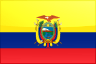# Ecuador Primary completion rate Stats

#### Definitions

• Female > % of relevant age group: Primary completion rate is the percentage of students completing the last year of primary school. It is calculated by taking the total number of students in the last grade of primary school, minus the number of repeaters in that grade, divided by the total number of children of official graduation age.
• Male > % of relevant age group: Primary completion rate is the percentage of students completing the last year of primary school. It is calculated by taking the total number of students in the last grade of primary school, minus the number of repeaters in that grade, divided by the total number of children of official graduation age.
• Total > % of relevant age group: Primary completion rate is the percentage of students completing the last year of primary school. It is calculated by taking the total number of students in the last grade of primary school, minus the number of repeaters in that grade, divided by the total number of children of official graduation age.
STAT AMOUNT DATE RANK HISTORY
Female > % of relevant age group 101.15% 2004 29th out of 132
Male > % of relevant age group 99.99% 2004 33th out of 132
Total > % of relevant age group 100.56% 2004 35th out of 140## APA MLA MHRA CSE AMA Chicago Bluebook Bluebook/JOLT Citation